# Letter Word Arrangement of Logical Reasoning

• Last Updated : 01 Jul, 2022

Q1. If it is possible to make a meaningful word from the 1st, 3rd, 6th, and 8th letters of the word “NOMINATION”, then which will be the 3rd letter of the word? Mark X if no such word can be formed, Y if more than one such word can be formed.
a) T
b) Y
c) I
d) S
e) X

Correct option – c
Analysis – 1st, 3rd, 6th, and 8th letters of the given word are N, M, A, I
So, the word formed is MAIN. Hence, the third letter of the word is I.

Q2. If it is possible to make a meaningful word from the 2nd, 5th, 6th, and 9th letters of the word “TRADEMARK”, then which will be the last letter of the word? Mark X if no such word can be formed, Y if more than one such word can be formed.
a) Y
b) L
c) V
d) E
e) X

Correct option – e
Analysis – 2nd, 5th, 6th, and 9th letters of the given word are R, E, M, K
So, no word can be formed. Hence, X is the correct answer.

Q3. If it is possible to make a meaningful word from the 2nd, 4th, 8th, 9th, and 13th letters of the word “DISADVANTAGES”, then which will be the 4th letter of the word from left? Mark X if no such word can be formed, Y if more than one such word can be formed.
a) I
b) N
c) T
d) A
e) X

Correct option – b
Analysis – 2nd, 4th, 8th, 9th and13th letters of the given word are I, A, N, T, S.
So, the word formed is SAINT. Hence, the 4th letter of the word is N. Therefore option (b)  is the correct answer.

Q4. If it is possible to make a meaningful word from the 2nd, 4th, 6th, and 7th letters of the word “STADIOMETER”, then which will be the first letter of the word? Mark J if no such word can be formed, K if more than one such word can be formed.
a) O
b) M
c) K
d) D
e) J

Correct option – e
Analysis –   2nd, 4th, 6th, and 7th letters of the given word are T, D, O, M
So, no word can be formed. Therefore, J is the correct answer.

Q5. If it is possible to make a meaningful word from the 1st, 5th, 6th, 10th & 13th letters of the word “INTERNATIONAL”, then which will be the 5th letter of the word? Mark X if no such word can be formed, Y if more than one such word can be formed.
a) R
b) O
c) L
d) N
e) X

Correct option – e
Analysis – 1st, 5th, 6th, 10th & 13th letters of the given word are I, R, N, O, L
So, no meaningful word can be formed. Hence, X is the correct answer.

Q6. If it is possible to make a meaningful word from the 1st, 2nd, 5th, 8th & 12th letters of the word “ASYMPTOMATIC”, then which will be the 1st letter of the word? Mark X if no such word can be formed, Y if more than one such word can be formed.
a) C
b) S
c) Y
d) A
e) X

Correct option – a
Analysis –  1st, 2nd, 5th, 8th & 12th letters of the given word are A, S, P, M, C
So, the word formed is CAMPS. Hence, the first letter of the word will be C.

Q7. If it is possible to make a meaningful word from the 2nd, 5th, 7th, 8th and 10th letters of the word “MANOEUVRING”, then which will be the 5th letter of the word? Mark J if no such word can be formed, Y if more than one such word can be formed.
a) N
b) V
c) A
d) E
e) J

Correct option – e
Analysis – 2nd, 5th, 7th, 8th & 10th letters of the given word are A, E, V, R, N
So, no meaningful word can be formed. Hence, J is the correct answer.

Q8. If it is possible to make a meaningful word from the 1st, 5th, 6th, 8th, and 11th letters of the word “ENVIRONMENTAL”, then which will be the 3rd letter of the word? Mark X if no such word can be formed, Y if more than one such word can be formed.
a) M
b) O
c) T
d) X
e) Y

Correct option – d
Analysis – 1st, 5th, 6th, 8th and11th letters of the given word are E, R, O, M, T
So, no meaningful word can be formed. Hence, X is the correct answer.

Q9. If it is possible to make a meaningful word from the 3rd, 5th, 9th, 10th, and 11th letters of the word “DELETERIOUS”, then which will be the 5th letter of the word from left? Mark J if no such word can be formed, K if more than one such word can be formed.
a) U
b) S
c) O
d) K
e) J

Correct option – b
Analysis – 3rd, 5th, 9th, 10th & 11th letters of the given word are L, T, O, U, S
So, the word formed is LOTUS. Hence, the 5th letter of the word is S.

Q10. If it is possible to make a meaningful word from the 1st, 6th, 8th, 9th and 10th letters of the word “SUBSCRIPTIONS”, then which will be the 1st letter of the word? Mark X if no such word can be formed, Y if more than one such word can be formed.
a) R
b) I
c) T
d) Y
e) X

Correct option – Y
Analysis – 1st, 6th, 8th, 9th and10th letters of the given word are S, R, P, T, I
So, the word formed is TRIPS, SPIRT, and STRIP. More than one word is formed. hence answer is Y

Q11. How many such letters are there in the word ‘STUDIO’ in which the letters are the same as in the English alphabet both from backward and forward directions?
a) None
b) Two
c)  More than three
d) Three
e) One

Correct option – d
Analysis –

So, there are three forward pairs – ST, TU, and  SU
Hence, option d) is the correct answer.

Q12. How many such letters are there in the word ‘NOURISHMENT’ in which the letters are the same as in the English alphabet both from backward and forward directions?
a) None
b) Two
c)  More than three
d) Three
e) One

Correct option – d
Analysis –

So, there are three forward pairs – NO, OS, and NS
Hence, option d) is the correct answer.

Q13. How many such letters are there in the word ‘KERATIN’ in which the letters are the same as in the English alphabet both from backward and forward directions?
a) None
b) Three
c)  Two
d) More than three
e) One

Correct option – b
Analysis –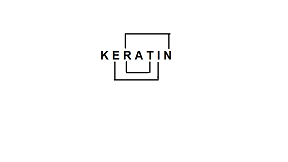So, there are two forward pairs – EI, RT
One backward pair – NR
Hence, option b) is the correct answer.

Q14. How many such letters are there in the word ‘DIVERSITY’ in which the letters are the same as in the English alphabet both from backward and forward directions?
a) None
b) Three
c)  Two
d) More than three
e) One

Correct option – c
Analysis –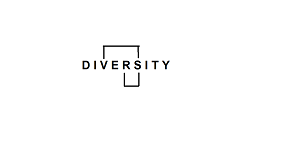So, there is one forward pair – RS
One backward pair – SV
Hence, option c) is the correct answer.

Q15. How many such letters are there in the word ‘CLONOGENIC’ in which the letters are the same as in the English alphabet both from backward and forward directions?
a) Five
b) Three
c)  Four
d) More than six
e) Six

Correct option – d
Analysis –

So, there are three forward pairs – LN, NO, and LO
Four backward pairs – CG, IN, NO, and IO
Hence, option d) is the correct answer.

Q16. How many such letters are there in the word ‘MOTILITY’ in which the letters are the same as in the English alphabet both from backward and forward directions?
a) None
b) Three
c)  Two
d) More than three
e) One

Correct option – b
Analysis –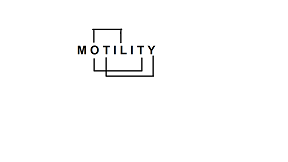So, there are two forward pairs – OT, TY
One backward pair -LO
Hence, option b) is the correct answer.

Q17. How many such letters are there in the word ‘GESTATION’ in which the letters are the same as in the English alphabet both from backward and forward directions?
a) None
b) Three
c)  Two
d) More than three
e) One

Correct option – c
Analysis –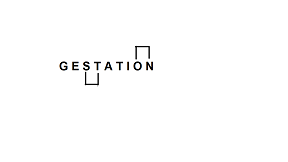So, there is one forward pair – ST
One backward pair – NO
Hence, option c) is the correct answer.

Q18. How many such letters are there in the word ‘DISTENDED’ in which the letters are the same as in the English alphabet both from backward and forward directions?
a) None
b) Two
c) More than three
d) Three
e) One

Correct option – c
Analysis –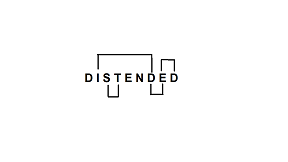So, there are two forward pairs – ST, DE
Two backward pairs – DE, DI
Hence, option c) is the correct answer.

Q19. How many such letters are there in the word ‘TRIDENT’ in which the letters are the same as in the English alphabet both from backward and forward directions?
a) None
b) Two
c) More than three
d) Three
e) One

Correct option – b
Analysis –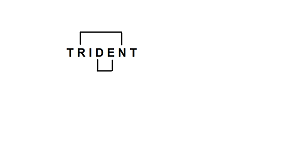So, there is one forward pair – DE
One backward pair – NR
Hence, option b) is the correct answer.

Q20. How many such letters are there in the word ‘RETRIEVE’ in which the letters are the same as in the English alphabet both from backward and forward directions?
a) None
b) Two
c) More than three
d) Three
e) One

Correct option – e
Analysis –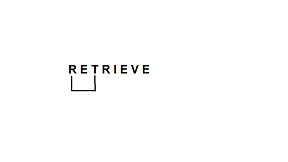So, there is one forward pair – RT
Hence, option e) is the correct answer.

My Personal Notes arrow_drop_up
Recommended Articles
Page :